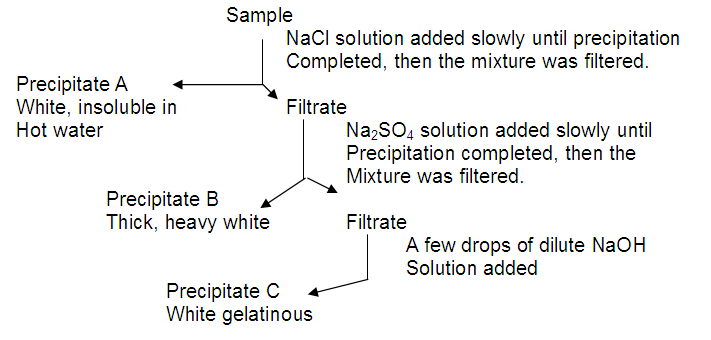CHEMISTRY

 Time: 1 Hour Max.Marks : 60

Instructions:

(1) Answer must be written either in English or the medium of instruction of the candidate in high school.

(2) There will be no negative marking

(3) Use of calculators or graph papers is not permitted

(4) There are TWENTY questions. Answer all the questions.

SECTION - A

Marks10x2=20

1. The reaction between dichromate ions and iron (II) ions can be carried in the presence of either dilute HCl (or) sulphuric acids. But the dilute HCl cannot be used to acidify the solution for titration between Iron (II) and permanganate ion system. The reason for this is ________.

2. Acetic acid is a weak acid and hydrochloric acid is a strong acid it follows that the

(A) pH of the 0.1M HCl will be 1

(B) Solution containing 0.1 mole acetic acid and 0.1 mole of sodium acetate will be a good buffer

(C) pH of the 0.1M HCl will be less than that of 0.1M acetic acid

(D) pH of a solution formed by mixing equimolar quantities of sodium hydroxide and HCl will be greater that of a similar solution formed from sodium hydroxide and acetic acid.

The correct statement(s) is/are ________________

3. A metal oxide has formula X2O3 it can be reduced by hydrogen to give free metal and water. 0.1596 g of metal oxide requires 6 mg of hydrogen for complete reduction. The atomic weight of the metal is __________.

4. Helium is mixed with the oxygen gas for deep sea divers. The percent by volume of oxygen gas in the mixture of the diver has to submerge to a depth where the total pressure is 4.2 atm and partial pressure of oxygen is maintained at 0.2 atm at this depth is ___________ .

5. In the reaction A + B ® products, if the concentration of B is kept constant and concentration of A is doubled, the rate becomes four times of initial rate and when A is kept constant and concentration of B is doubled rate remains the same. This indicates that reaction rate is proportional to the __________ of concentration of ___________ and rate equation is _____________.

6. One ________ of a substance is equal to its atomic or molecular mass expressed in __________ and contains 6.023x1023 chemical particles, which is known as ______________ of particles. Since 1 mole of any chemical substance contains same number __________ as 1 mole of any other chemical substance, we can calculate the weight of one chemical that will react with a given weight of other by using their _________ in other calculations.

7. 15 moles of H2 and 5.2 moles of I2 are mixed and allowed to attain equilibrium. Concentration of HI is found to be 10 moles the equilibrium constant for the formation of HI is ____________.

8. 600 ml of a mixture of O2 and O3 weighs 1 gram at NTP. The volume of ozone in the mixture _______.

9. At 27oC 4.6 grams of N2O4 undergoes decomposition and exerts an equilibrium pressure of 1.6 atmosphere when vapourised in a 1 lit flask. The fraction of N2O4 dissociated is ________. [N2O4(g) 2NO2(g)]

10. A compound contains 28% nitrogen and 72% metal by mass. 3 atoms of the metal combine with 2 atoms of the nitrogen. The atomic weight of the metal is __________

Marks 5x1=5

11. The V.D of Gas is 15. 15 g of this gas will occupy a volume of ______ cc at 273oC and 1 atm.

12. You are given 500 ml of 2N HCl and 500 ml of 5N HCl. The maximum volume of the 3 M that you can make from these two solutions is ___________.

13. The equilibrium constant for the reaction 2A + 2B 2C + 2D is 100. The equilibrium constant for the A + B C + D at same temperature is _________ .

14. The solubility product of sparingly soluble salt Ag2CrO4 is 4x10-12. The molar solubility of the salt is _____________

15. For the preparation of HBr in the lab the corresponding bromide salt should be treated with __________ acid.

SECTION - B

Marks 10x2=20

16. Differentiate the following:

(a) Redox reaction – disproportionation reaction

(b) Primary standard substance – Secondary standard substance

(c) Electrolysis – Hydrolysis

(d) Gangue – Slag

(e) Lattice energy – Hydration energy

(f) Equilibrium constant – Rate constant

(g) Ionic product – Solubility product

(h) Activation energy – Threshold energy

(i) Valence – Oxidation number

(j) Allotropy – Enantiotropy

SECTION - C

17. A compound of oxygen and nitrogen has the composition 1.52 g N and 3.47 g of oxygen. The molar mass of this compound is known to be between 90 and 95 g. Determine the molecular formula and molecular mass of compound. 3M

18. A transition metal X forms an oxide of formula X2O3. It is found that only 50% of X atoms in this compound are in the +3 oxidation state. The only other stable oxidation states of X are +2 and +5. What percentage of X atoms are in the +2 oxidation state in this compound? 4M

19. Many diabetics must determine their blood sugar content daily. The measurements provided by the devices used for such purpose are in milligrams per deciliter. 3M

(a) What is the concentration of glucose [C6H12O6] in grams per liter when a reading of 95 is observed?

(b) What is the concentration in moles per litre?

20. A student was given a sample of colourless solution containing three cations and was asked to identify the cations. The student carried out a series of reactions as shown in the flow chart below. 5M(i) From the information above, suggest three cations which could be in the sample and would form the precipitates A, B and C. Give the formula for each precipitate,

(ii) Give an additional test which would help to confirm the presence of each cation,

(iii) Name any other cations that would fit the requirements for precipitates A, B and C.